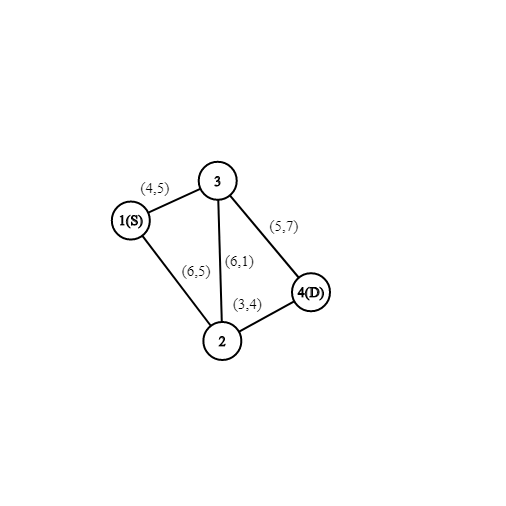InterviewBit Academy is now Scaler Academy!# Rust and his transfer

Detective Rust is being transferred to a new station across the country and has a limited relocation bonus to get him there. In addition to the relocation bonus, he is entitled to one additional luxury taxi ride between any two cities en route his destination. He may or may not choose to travel by the taxi.

Rust wonders about the most optimal way (in terms of time of cost) of traveling from his originating station C to his new station D with or without using his entitled taxi ride. The intercity map is given as a graph with A nodes (labeled from 1 to A). There are undirected edges between each of the given nodes with two costs; one denotes the cost of a path using Rust’s own mode of travel, and the other denotes the cost of a taxi between a pair of cities.

Help Rust minimize the cost of his move!

Input Format:

``````    First argument of input contains a single integer A denoting number of nodes.
Second argument of input contains a M x 4 matrix B denoting undirected road. First and second integer are starting and ending node, third integer is regular travel cost and fourth integer is taxi cost.
Third argument of input contains a single integer C denoting Rust starting station.
Fourth argument of input contains a single integer D denoting Rust destination station.
``````

Output Format:

``````    return a single integer denoting minimum cost. if destination is unreachable return -1 instead.
``````

Constraints:

``````    2 <= A <= 3000
1 <= M <= min(A*(A-1),1000000)
1 <= B,B,C,D <= A
1 <= B,B <= 500
``````

For Example:

``````Input 1:
A = 4, B = [[1, 2, 6, 5], [1, 3, 4, 5], [2, 3, 6, 1], [2, 4, 3, 4], [3, 4, 5, 7]], C = 1, D = 4
Output 1:
8
Explanation:
Optimum route would be 1 => 2 -> 4
Input 2:
A = 3, B = [[1, 3, 5, 50]], C = 1, D = 2
Output 2:
-1
``````NOTE: You only need to implement the given function. Do not read input, instead use the arguments to the function. Do not print the output, instead return values as specified. Still have a doubt? Checkout Sample Codes for more details.